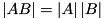# Matrix Determinant

Given a matrix,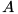, its determinant can be written as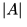or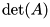.

A determinant can only be calculated from a square matrix.

The determinant of a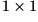matrix: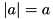The determinant of a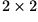matrix: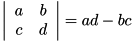The determinant of a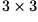matrix: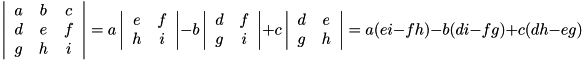More generally, to calculate the determinant of a matrix, for example: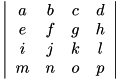Take the first row: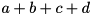Make every second element negative: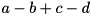For each element, ignore the row and column it's in: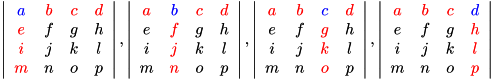And multiply it by the determinant of the remaining elements: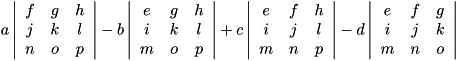Then recursively decompose the resulting determinants until you're left with the trivial case of a single-element matrix.

(the above method applies toandmatrices, but it helps to have a bigger matrix for demonstration)

Determinants have the property that: